# Miscellaneous Solved Problems - LabVIEW

Rating: 4

6200

## Miscellaneous Solved Problems

1.Check controls and indicators:
Numeric Control and Indicator

• Boolean Control and Indicator
• String Control and Indicator
• Dial connected to Gauge and Meter
• Thermometer to Numeric Indicator
• Tank to Numeric Indicator
• Vertical Slide Control to Horizontal Slide Control

If you would like to Enrich your career with an Android certified professional, then visit Mindmajix - A Global online training platform: “LabVIEW training” Course.This course will help you to achieve excellence in this domain.

Solution:The front panel with controls and indicators and the respective block diagrams are shown in Figures P2.1(a) and P2.1(b).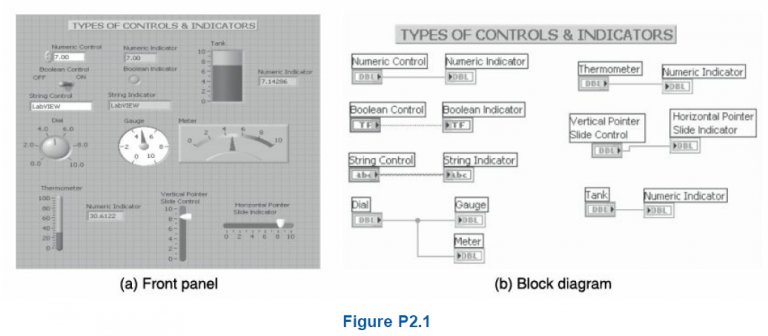2.Add, multiply, subtract and divide two numeric inputs.
Solution: The front panel has controls A and B and four indicators as shown in Figure P2.2(a). The block diagram has the respective terminals and functions as shown in Figure P2.2(b).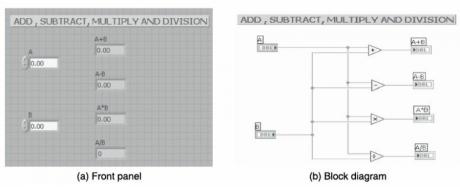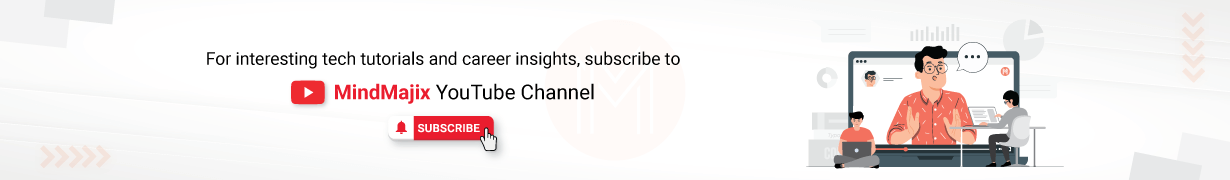3.Add and multiply more than two numeric inputs.
Solution: The front panel has three numeric inputs while the block diagram contains compound arithmetic functions as shown in Figures P2.3(a) and P2.3(b).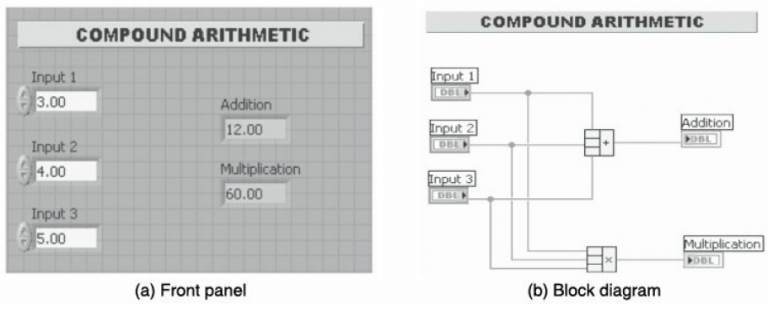4.Divide two numbers and find the remainder and quotient.
Solution: The front panel and block diagram for finding the remainder and quotient are shown in Figures P2.4(a) and P2.4(b).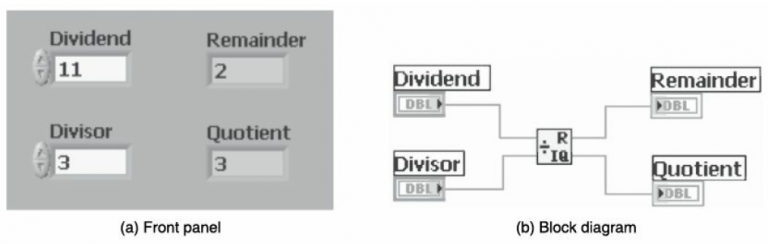5.Compute the expressions Y = (A*B*C) + (D*E) and Y = mx + c.
Solution: The front panel and block diagram for solving two equations are shown in
Figures P2.5(a) and P2.5(b).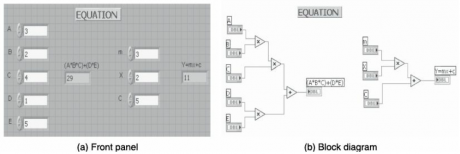6.Compute the equations (X1+2)*log(X1) using functions, Expression node and Express Formula for the given inputs X1.
Solution: The front panel and block diagram for solving an equation using three different ways are shown in Figures P2.6(a) and P2.6(b).

Check Out LabVIEW Tutorials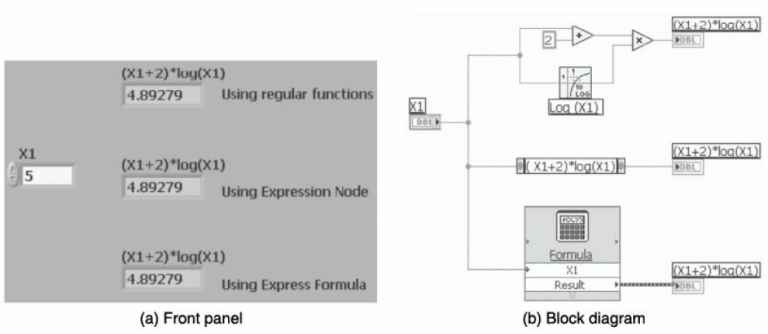7.Convert Celsius to Fahrenheit.
Solution The front panel and block diagram for solving the equation F = (1.8C + 32) are shown in Figures P2.7(a) and P2.7(b).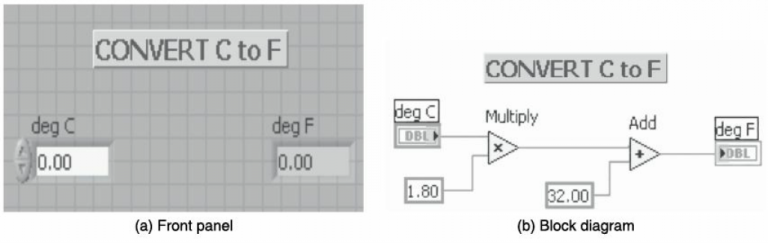8.Find whether the given number is odd or even.
Solution: Click continuous run and increment the number to see the even and odd LED glow alternatively. The front panel and block diagram are shown in Figures P2.8(a) and P2.8(b).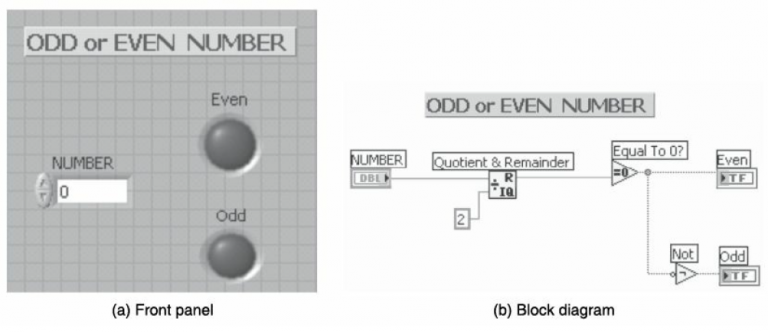Solution: The front panel and block diagram are shown in Figures P2.9(a) and P2.9(b) where Degrees = Radians (180/p).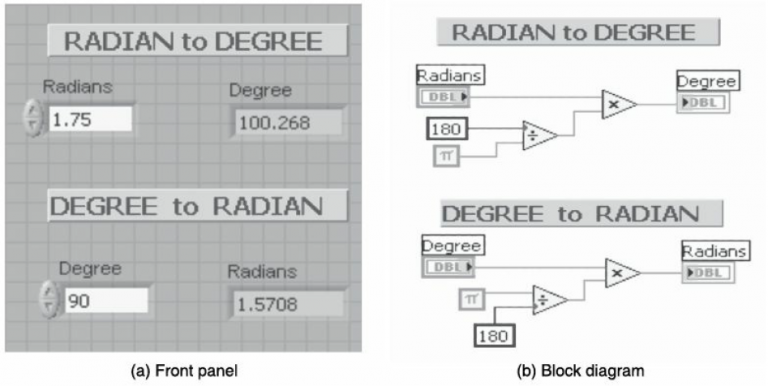10.Find trigonometric values for the given angle. In LabVIEW computation of trigonometric values such as sine, cosine and tangent takes the input in radians. Hence, angles in degrees are to be converted to radians before calculation.
Solution: The angle is provided as control and the corresponding radians, sin, cos and tan values are displayed. The front panel and block diagram are shown in Figures P2.10(a) and P2.10(b).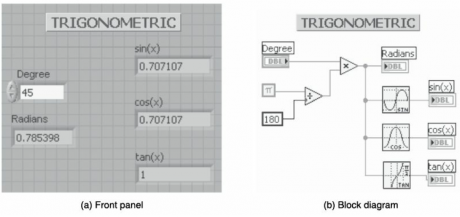11.Perform various Boolean Operations (AND, OR, NAND, NOR, XOR).
Solution: The front panel and block diagram are shown in Figures P2.11(a) and P2.11(b) and the truth table for Boolean operations can be checked.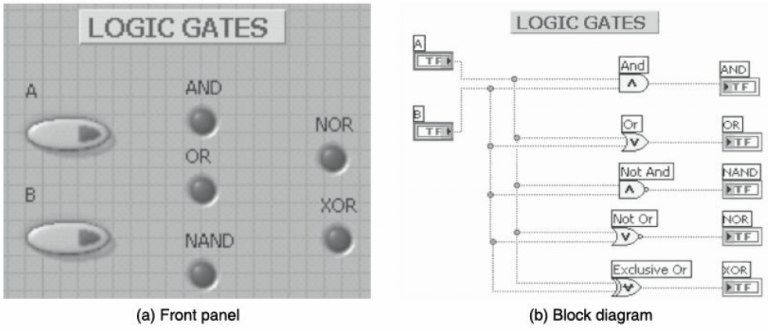12.Convert a binary number to a decimal number.
Solution: The front panel and block diagram are shown in Figures P2.12(a) and P2.12(b) to convert a binary to a digital number.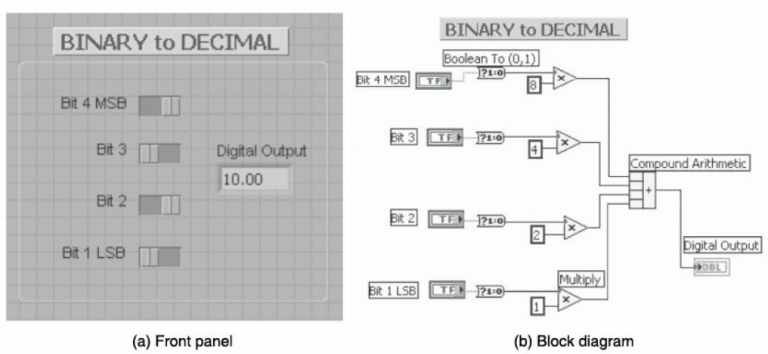13.Split an input string into two outputs with reference to a separating character. Find the length of the input string and reverse the string.
Solution: Any input string can be provided. In this problem “LABVIEW BASICS” is given and the front panel and block diagram are shown in Figures P2.13(a) and P2.13(b).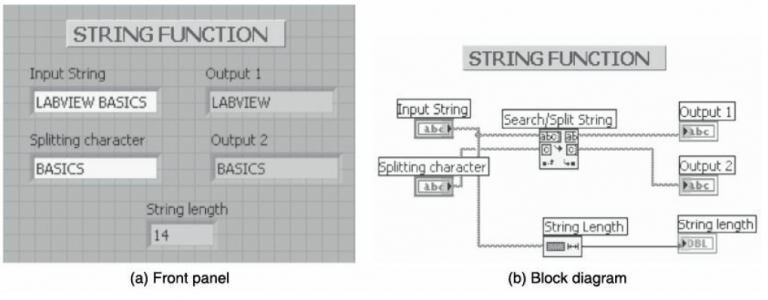14.Add two binary bits and find the sum and carry (half adder).
Solution: The front panel and block diagram are shown in Figures P2.14(a) and P2.14(b) and the half adder can be checked.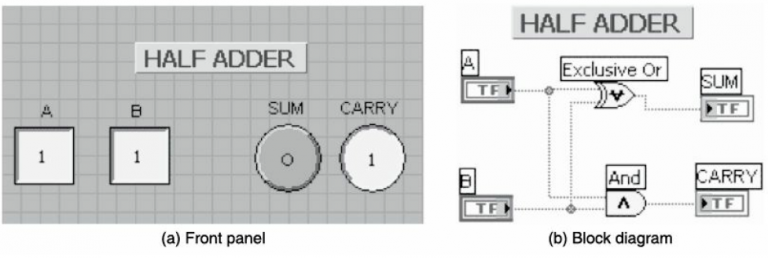Course Schedule
NameDates
LabVIEW TrainingFeb 11 to Feb 26
LabVIEW TrainingFeb 14 to Mar 01
LabVIEW TrainingFeb 18 to Mar 05
LabVIEW TrainingFeb 21 to Mar 08
Last updated: 08 February 2023